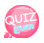# Operator

This time, let's talk about operators.

### Logical operator

Logical operators indicate whether they are true or false.

#### Kinds

AND(&,&&)

OR(|,||)

XOR(^)

The AND operator spits true when all the values ​​are true.

The OR operator spits out true if any of the values ​​are true.

The XOR operator spits true if they have different values, and false if they have the same value.

The table below makes it easy to understand.

 A B AND (&, &&) OR (|, ||) XOR(^) 0 (false) 0 (false) 0 (false) 0 (false) 0 (false) 0 (false) 1 (true) 0 (false) 1 (true) 1 (true) 1 (true) 0 (false) 0 (false) 1 (true) 1 (true) 1 (true) 1 (true) 1 (true) 1 (true) 0 (false)

In the table above, when A is false, B is false, AND operator tells false, OR and false XOR also tells false.

The reason is that the AND operator tells true when both are true, the OR tells true if any one is true, and the XOR tells true when they are different.

### Increment and Decrement Operators

There are two types of increment operators.

There are ++ for increasing and-for decreasing.

Each one increases or decreases one by one.

Suppose there is a variable called a

The difference is when you place it before or after it.

For example

++a, a++, --a, a--

I use it as above.

++ a first multiplies itself and starts another operation

a ++ first does another operation and then increments itself by one.

Let's make a comparison through the source.

```<!DOCTYPE html>
<html>
<title>JavaScript</title>
<script>
a = 10;
b = ++a;
document.write(b);
</script>
<body>
</body>
</html>```

### Source description

Explaining the above source, I assigned 10 to a and then ++ a to b.

```<!DOCTYPE html>
<html>
<title>JavaScript</title>
<script>
a = 10;
b = a++;
document.write(b);
</script>
<body>
</body>
</html>```

### Source Description

If you explain the source above, you assign a to 10 and then a ++ to b.
If ++ is followed, we first do another operation and increment itself by one.
10, the value of a, is assigned to b first and then increases itself by 1. therefore
Therefore, the result is 10.

### Logic negation (!)

Logical negation! The operator converts true to false and false to true.
So let's look at the following example to understand.

```<!DOCTYPE html>
<html>
<title>JavaScript</title>
<script>
a = true;
b = !a;
document.write(b);
</script>
<body>
</body>
</html>```

### Source Description

The source above assigns true to a. It is not a string and is not surrounded by "".
And we assigned! A to the variable b. ! Reverses the value, so a that was true is assigned to b after it becomes false. Therefore, the value of b is false.

### Relational operator

The types of relational operators are as follows:

&lgt;Great.
<= Greater than or equal to
> Small
> Less than or equal
== equals (===)
!= Not equal (!==)
In other words, if there is an expression 3 <4, false is outputted.
And if there is an expression 4 <3, true is outputted.
There are equals == and ===. If they are the same, it shows true.
There is! = And! == for not equals. True if they are not equal.
What is the difference between == and === and what is the difference between! = And! ==?
== is true if the values ​​are equal, but === must be equal to both the value and the type.
! = Is true if the values ​​are different, but! == is true if the types are different and the values ​​are different.
Let's check it using various expressions.

```<!DOCTYPE html>
<html>
<title>JavaScript</title>
<script>
document.write("10==1 is"+(10==1));
document.write("<br />");
document.write("<br />");
document.write("10!=10 is"+(10!=10));
document.write("<br />");
document.write("<br />");
document.write("20>40 is"+(20>40));
document.write("<br />");
document.write("<br />");
document.write("20<40 is"+(20<40));
document.write("<br />");
document.write("<br />");
document.write("50>=40 is"+(50>=40));
document.write("<br />");
document.write("<br />");
document.write("20<=20 is"+(20>=20));
document.write("<br />");
document.write("<br />");
document.write("40===40 is"+(40===40));
document.write("<br />");
document.write("<br />");
document.write("'40'===40 is"+('40'===40));
document.write("<br />");
document.write("<br />");
document.write("40==40 is"+(40==40));
document.write("<br />");
document.write("<br />");
document.write("'40'==40 is"+('40'==40));
document.write("<br />");
document.write("<br />");
document.write("30!==40 is"+(30!==40));
document.write("<br />");
document.write("<br />");
document.write("'30'!==40 is"+('30'!==40));
document.write("<br />");
document.write("<br />");
document.write("30!=40 is"+(30!=40));
document.write("<br />");
document.write("<br />");
document.write("'30'!=40 is"+('30'!=40));
document.write("<br />");
document.write("<br />");
document.write("30!==40 is"+('40'!==40));
</script>
<body>
</body>
</html>```

### Conditional operator

#### Variable = condition? True: false;

Variable = condition? True: false; If the condition is true, it assigns true; if false, it assigns false to the variable.
For example,
number = 10> 5? "number": "string";
In the above expression, if 10> 5 is large, assign the string number to the variable number, otherwise assign the string string. Since 10 is greater than 5, you assign the string number to the variable number.
Another example
number = 10> 5? 10: 5;
The above expression assigns 10 to number or 5 if 10 is greater than 5. Now you know?
Practice as you type.

```<!DOCTYPE html>
<html>